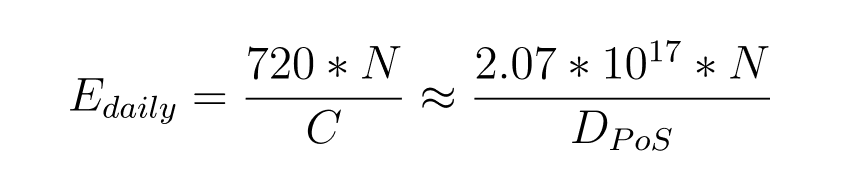# Estimating PoS earnings

Proof-of-stake earnings depend on current PoS difficulty and the number of coins you have locked for staking. The more coins you stake, the better chance you have to “win” the right to sign the next PoS block. Of course, it’s important to estimate your chances and predict how much you can earn in some way. Here is the most straightforward way to do so.

First, we need to get the current PoS difficulty, which can be found in the block explorer. This value is then divided by 1000000000000 (10 ^ 12), which in the Zano network is one coin basis.

Second, we again divide it by `288`. This operation lets us take the PoS mining timestamp frame into account. Without further details, this factor provides hash variety in PoS mining and can be somewhere between 256 and 320. It’s reasonable to use the value of 288 here.

Now we have an estimation of how many coins participate in PoS mining currently as
`Coins_in_PoS = PoS_difficulty / 1000000000000 / 288` or:where `C` is the total amount of coins participating in PoS mining, and `D_PoS` is the current PoS difficulty.

As you may know, the Zano network emits an average of 1 coin each minute with a 50-50 spread between PoS and PoW. That makes it `720` potential PoS reward coins per day. So if you owned all the coins in PoS, that could be your total day earnings. And if you divide `C` by `720`, you will get the number of coins you need to mine 1 Zano coin a day. Now, you can estimate the number of coins you will earn as `your_PoS_earnings = 720 * your_staking_coins / C` or, in other words:where `E_daily` is the estimated number of coins you'll earn per day, and `N` is the number of coins you're staking.

Please keep in mind that all the above is an expectation and can vary heavily.